## Introduction

### Name

LISP is an acronym for "LISt Processing Language" - so named because the list is one of the primary data structures in the language. Symbolic AI regards symbolic lists as being a key part of the way intelligent beings and systems actually store and manipulate information.

### History

LISP is the second oldest higher-level language (after FORTRAN), first developed by John McCarthy (then of MIT) following the Dartmouth Conference of 1956 that is often taken as the birth of the discipline of Artificial Intelligence. Though it has been used in other areas, it is primarily identified with the field of AI and was the "lingua franca" of AI work for many years (arguably still so).

In LISP's early years, mutually incompatibile dialects proliferated as it was implemented on different platforms. In 1984, a standardization effort resulted in the development of Common Lisp, as defined in Guy Steele, Common Lisp: The Language. (The second edition of this book remains one of the best-selling LISP books on amazon.com's ranking). Common Lisp became an ANSI standard in 1994. Today, most implementations of LISP attempt to adhere to the Common Lisp standard.

One early dialect of LISP - Scheme - has survived to the present day, and is also a standardized and widely used language. While it resembles Common Lisp, it also differs from it in a few ways that cause some to regard it as a distinct language. At the same time, some ideas that were pioneered in Scheme (e.g. static versus dynamic scoping of names) also found their way into Common Lisp - not surprising given that the same individual (Guy Steele) was a co-creator of Scheme as well as the author of the definition for Common Lisp. This page will discuss Common Lisp.

### Resources

Two important books on LISP have been made freely available by their publishers on the Internet:

## Basic Concepts

### LISP Belongs to the Functional Language Paradigm

LISP is the earliest representative of the functional programming language paradigm. Unlike procedural and object-oriented languages - whose theoretical model of computation is the Turing Machine, LISP's theoretical model of computation is the Lambda calculus developed by Alonzo Church. (It can be shown that the two models of computation are equivalent in power - that is, any algorithm that can be expressed in one model can also be expressed in the other).

This difference might be understood in terms of the following diagram: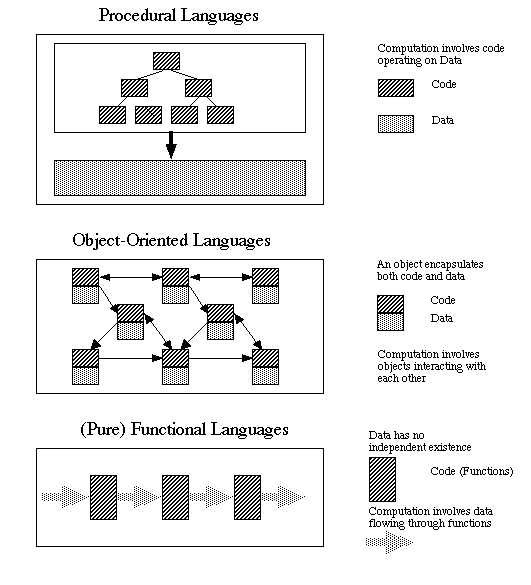In procedural languages, code operates on data; in object-oriented languages, objects encapsulate code and data and interact with one another; in functional languages data flows through functions but has no separate existence of its own. That is, in procedural languages data is passive; in OO languages it is active; in pure functional languages it is ephemeral.

However, most functional languages (including LISP) provide a mechanism for keeping certain data in existence even when it is not flowing through functions. In LISP, this takes the form of what Common Lisp calls "special variables" - essentially equivalent to what in other programming languages are called "global variables". That is to say, though LISP is a functional language, it is not a pure functional language (though it could be used as one by avoiding imperative constructs.)

### LISP Uses a Variant of Prefix Notation

LISP uses a variant of prefix (Polish) notation called Cambridge Polish Notation. All expressions are fully parenthesized, with the first element of an executable form being the name of a function, macro, or special form. For example, the expression written as 1 + 2 * 3 - 4 in traditional infix notation is written as `(- (+ 1 (* 2 3)) 4)` in LISP.

### Representation of Information

A further critical characteristic of LISP - which most functional languages do not share, is that there is absolutely no syntactic distinction between functions and data. For example, in one context, ```(car '(volkswagen golf))``` may be a piece of data representing a particular motor vehicle. In another context, it may be taken as the application of the function `car` to the list `(volkswagen golf)`. (It turns out that, since `car` is a defined operation in LISP, this form is meaningful and has the value `volkswagen`!)

In McCarthy's original paper, data objects were referred to as s-expressions (symbolic expressions). Common Lisp defines a rather complex hierarchy of data types; however, there are only a few core types of s-expression that have been part of the language since the beginning. These include:

• Symbols

LISP symbols correspond to what other programming languages typically call identifiers - though in LISP the rules for forming symbols are much less restrictive than the rules for forming identifiers in other languages. For example, all of the following are valid symbols:
```	word
this-is-a-symbol
*this-is-also-a-symbol*
123so-is-this
```
LISP is not case sensitive, so, for example, `word`, `Word`, `WORD`, and for that matter `wOrD` are all equivalent.

Along with numbers, symbols are also called atoms.

• Numbers

The LISP notion of a number is similar to that in other languages, though Common LISP recognizes a wide variety of types of number with distinct representations (e.g. ratios, complex numbers ...). For example, all of the following are valid numbers, with meanings the same as in most languages:
```	42
3.14
6.02e23
```
Along with symbols, numbers are also called atoms.

• (True) Lists

A LISP list consists of any number of s-expressions separated by one or more spaces and enclosed in parentheses. For example, all the following are valid lists:
```	(a list)
(a list (that contains another list))
((small prime numbers) (2 3 5 7))
```

• The Empty List

A list that contains no elements ( `()` ) is a legitimate list. It has an alternate name - `nil`. These two names mean exactly the same thing - though one may prefer to use one name or the other depending on the context. The empty list ( `()` or `nil` ) has the interesting property that it is the one and only s-expression that is both a symbol (and hence an atom) and a list.

• Conses (Dotted Pairs)

A dotted pair consists of exactly two s-expressions enclosed in parentheses and separated by a dot. For example, all the following are valid dotted pairs:
```	(1 . 2)
(a . b)
(this . (is . (another . (way . (of . (representing . (a . (list . nil))))))))
```
Dotted pairs do not appear often in LISP programs, but as the last example indicated, they are actually the way that LISP represents lists internally. For instance, the last example above is not a true list (though it may be referred to as a "dotted list"; however, it is equivalent to the list `(this is another way of representing a list)`. Note well that the last dotted pair in the internal representation of a true list consists of the last element and the empty list (`nil`) - it is not a dotted pair consisting of the last two elements! For example, `(this . (is . (not . (what (you . think)))))` is not the true list `(this is not what you think)` but rather the dotted list `(this is not what (you . think))`!

It is sometimes helpful to picture the way that LISP represents a list in terms of dotted pairs (conses). For example, the list `(this is a list)` is represented internally as `(this . (is . (a . (list . nil))))`, which looks like this in memory: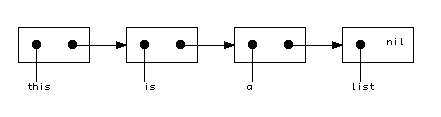The following is the complete type hierarchy of Common Lisp. Note well that every s-expression is either an atom or a list, except for `()` / `nil`, which is both!

Common Lisp type name Printed form example(s)
Atom
Symbol`x`, `this-is-a-symbol`, `*so-is-this*`, `1+`, `nil`, `()`
Keyword`:test`
Number
Integer
Fixnum`123`, `-4`, `#o377` (octal), `#b10100011` (binary)
Bignum`12345678901234567890`,
Ratio`4/5`
Float
Short-Float`-1.3s-4`
Single-Float`-1.3e-4`, `.00013`
Double-Float`-1.3d-4`
Long-Float`-1.3l-4`
Complex`#c(3.2 4.0)`
Character`#\A`, `#\a`, `#\Control-A` (all 3 different as characters)
Bit`0`, `1` (printed only, cannot be read)
Vector`#(1 2 3)`, `#(apples bananas cumquats)`
Bit-Vector`#*101010`
String`"This is a string"`
Array`#2a((1 2 3) (4 5 6))`
Structure```#s(student-info :first-name john :last-name jones :gpa 2.37)```
(valid only if the structure `student-info` has been defined by `defstruct`)
List
True List```(1 2 3), (), nil```
Dotted List`(1 . 2)`,   `(1 2 (3 . 4))`

## Variables and Expressions

### Variables

A symbol (other than one of the self-evaluating ones listed below) is regarded as the name of a variable when it is evaluated. LISP is a dynamically typed language - a variable does not have a declared type, but simply takes on the type of its current value. Common Lisp recognizes two kinds of variables.

• Lexical variables are meaningful only in the context in which defined. Parameters of a function are lexical variables. So are variables bound by special forms like `let` or `let*`; the latter are analogous to local variables in other programming languages. Example:
```	(defun f (x)
(let ((y 3))
;; At this point, x and y are lexical variables
)
)
```
• Special variables are analogous to global variables in other programming languages. A special variable is usable in any context, unless it is shadowed by a lexical variable in some scope. A special variable is normally created and/or assigned a value by the special form `setq`. Example (assuming no lexical variable named `x` is in scope):
```	(setq x 3)
```
Creates the special variable `x` if necessary, and in any case gives it the value `3`.

Of course, if one is trying to program in functional style, the use of special variables is to be avoided!

Common Lisp uses two separate namespaces for the use of a symbol as a special form / macro / function name and the use of a symbol as a variable name. Thus, for example, the following is legal (though arguably a source of confusion)

```(setq setq 3)
```
This would cause the variable whose name is `setq` to be given the value `3`. When the LISP interpreter sees the name `setq` as the first element of a list to be evaluated, it takes it as the name of a special form; but any time the LISP interpreter sees a symbol like `setq` elsewhere, it takes it as the name of a variable. (This turns out to be one of the significant differences between LISP and Scheme; the latter has only a single namespace, so a given symbol cannot be both the name of a variable and the name of a special form / macro / function.

### Expressions

In LISP, code takes the form of expressions to be evaluated. For example, `(+ 3 4)` is an expression that calls for applying the addition operation to the two numbers 3 and 4, yielding the result 7. We noted earlier that LISP makes absolutely no syntactic difference between data and code. As a result, any LISP s-expression might potentially be treated as an expression to be evaluated - which may or may not be meaningful. For example, we could attempt to evaluate `(3 4 5)` - but the result would be an error since this s-expression is not meaningful when viewed as code.

When attempting to evaluate a form, LISP uses the following rules:

• Some forms are self-evaluating - that is, the result of evaluating the form is the form itself. The self-evaluating forms are:

• The symbols `nil` (or `()`) and `t` (which is often used as the logical value true.)
• Keywords (symbols whose name begins with `:`)
• All kinds of numbers
• Characters
• Strings
• Bit-vectors

• Symbols (other than `nil`, `t`, and keywords) are treated as the names of variables. (See discussion above) If a symbol has been defined as the name of a lexical or special variable, the variable's value is its value; otherwise, attempting to evaluate it results in an error.

• Lists whose first element is a symbol are treated as the application of a function, special form, or macro (whose name is specified by the initial symbol) to the remainder of the elements treated as arguments. For example, `(+ 3 4)` is treated as the application of the function named by `+` to the arguments `3` and `4`, with the result (7) being the value of the expression.

• The set of special forms is defined as part of the language. A program cannot define new special forms or redefine existing ones.
• The topic of macros is beyond the scope of this page. In Common Lisp, there are a large number of pre-defined macros that behave in many ways like special forms. For the purposes of this page, we will not attempt to distinguish between the two - thus, we will discuss some pre-defined macros as if they were special forms (which they often were in earlier dialects of LISP.) It is also possible for the programmer to define new macros, but we will not discuss this.
• Common Lisp includes a large number of pre-defined functions. The programmer can define new functions, and can also redefine pre-defined functions (though this is not generally recommended). We will consider just a few pre-defined functions here.

• Attempting to evaluate any other type of s-expression is an error.

### Evaluating Functions

As noted above, any list whose first element is a symbol is regarded as either the application of a function or of a special form or macro to the remaining elements of the lists, regarded as its arguments. The LISP interpreter handles these quite differently, though.

When a function call is evaluated, the interpreter first evaluates each of the arguments in the list, and then applies the specified function to the result.

Example: consider the following form:
```(+ (* 2 3) (* 4 5)))
```
When evaluating it, the interpreter first evaluates the form `(* 2 3)`, then the form `(* 4 5)`. To evaluate `(* 2 3)`, it first evaluates `2` and then `3`. Since numbers are self-evaluating forms, their values are 2 and 3, and when the interpeter applies `*` to these evaluated arguments, the result is 6. Something similar happens when `(* 4 5)` is evaluated to yield 20. Then the function `+` is applied to these evaluated arguments 6 and 20, yielding the final result 26.

Example: consider the following code excerpt:

```(setq a 4)
(setq b 5)
(+ a b)
```

When evaluating `(+ a b)`, the interpreter first evaluates the forms `a` and `b`. Each is taken as the name of a variable, and the most recent values assigned to those variables (4 and 5) are used. Finally, the interpreter applies the function `+` to these values, yielding 9.

### Evaluating Special Forms and Macros

If the first symbol in a list to be evaluated is the name of a special form or macro, the interpreter does not evaluate the arguments. However, the special form or macro may evaluate some of the arguments - how this is handled varies from special form / macro to special form / macro. For example, the special form `setq` evaluates its second argument, but not its first.

Example: consider the second line of the following code excerpt:

```(setq a 1)
(setq a (+ 2 3))
```
Because the special form `setq` evaluates its second argument, the form `(+ 2 3)` is evaluated, yielding 5. However, the form `a` is not evaluated - so 5 is assigned to `a`. (If `setq` were a function or a special form that evaluated both of its arguments, the operation would mean that 5 should be assigned to 1 - obviously an absurd operation!)

## Some Common Lisp Special Forms, Macros, and Functions

The following are but a few of the special forms, macros, and functions of Common Lisp.

### Some Special Forms and Macros

• `quote`

`quote` is a special form that simply passes its unevaluated argument through. For example, the value of `(quote a)` is the symbol a.

As a convenience, the LISP reader considers the an expression of the form `'anything` to be equivalent to `(quote anything)`.

• `setq`

As discussed above `setq` is a special form that evaluates its second argument and then assigns the value to the variable named by its first argument.

• `let`

`let` is a special form that creates lexical variables. It has the following basic form (though variations are possible)

```	(let ( { (variable expression) }* ) { form }* )
```
The forms are evaluated in a context in which each of the variables is a lexical variable bound to the initial value specified. All of the expressions are evaluated before any of the variables is bound - therefore an expression cannot use a variable previously declared in the same `let`.

• `if`

`if` is a special form that selects one of two expressions to evaluate based on the outcome of some test, with its value becoming the value of the whole expression. It is analogous the conditional expression of C-family languages (```test ? then-expr : else-expr```), and has the following syntax
```	(if test then-form else-form)
```
The two forms must be single s-expressions; however `progn` can be used to group multiple forms (analogous to `{ ... }` in Java), though this is, of course, not purely functional style. The else-form may be omitted; in this case, if the test is false the result is `nil`.

• `cond`

`cond` is a macro that selects one series of expressions out of several to evaluate. It has the following basic form (though variations are possible)

```	(cond { ( test { form }*) }* )
```
Each test is evaluated in turn until one returns any result other than `nil` - at which point the following form(s) is/are evaluated. The value of the last form evaluated becomes the value of the `cond` - hence if there is more than one form, any form save the last is evaluated only for its side effects. It is common to see that the last clause of `cond` has the test `t`, which of course is always true and constitutes a default case. (Of course, if one is programming in pure functional form, each clause of a `cond` will have exactly one form.)

• `and` - macro with any number of arguments, which are usually all tests - true if all are non-nil - false if any is `nil`. Does short-circuit evaluation - i.e. stops evaluating arguments as soon as one that is false (`nil`) is encountered.

• `or` - macro with any number of arguments, which are usually all tests - true if any is non-nil - false if all are `nil`. Does short-circuit evaluation - i.e. stops evaluating arguments as soon as one that is true (non-nil) is encountered.

• `defun` - macro for defining named functions. The basic syntax is
```	(defun name ( { parameter }* ) { form }* )
```
When the function is called, the form(s) constituting the body are evaluated in sequence in a context where any parameter(s) are lexical variables bound to the actual parameters of the call; if there are multiple form(s) the value of the last becomes the value of the function and the others are evaluated only for side-effects. (Of course, if one is programming in pure functional style, the body of a function will consist of exactly one form.)

• `lambda` - macro for defining anonymous functions. The basic syntax is
```	(lambda  ( { parameter }* ) { form }* )
```
When the function is called, the form(s) constituting the body are evaluated in sequence in a context where any parameter(s) are lexical variables bound to the actual parameters of the call; if there are multiple form(s) the value of the last becomes the value of the function and the others are evaluated only for side-effects. (Of course, if one is programming in pure functional style, the body of a function will consist of exactly one form.)

### List Operations

The list operations are often best understood by picturing what they do in diagrammtic form, as done below. All are functions, which means that the arguments are evaluated before the operation is performed.

• `cons` - 2 arguments of any type. Constructs a dotted pair (cons cell) consisting of the 2 arguments. For example, `(cons 'a 3)` is `(a . 3)`• `car` - 1 argument, which must be a true or dotted list. Extracts the car of the first cell of the list. For example, `(car '(a 3))` is `a`• `cdr` - 1 argument, which must be a true or dotted list. Extracts the cdr of the first cell of the list. If the list consists of more than one cell, its cdr will itself be a list. For example, `(cdr '(a 3))` is `(3)`. (Note that this is a two element true list, as compared to a dotted pair as in the `cons` example.)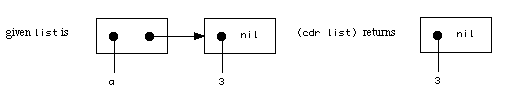• `caar`, `cdar`, `cadr`, `cddr`, `caddr`, `cdddr` and several more - any combination of up to four a's or d's - convenience forms equivalent to `(car (car ...))`, `(cdr (car ...))`, `(car (cdr ...))`, `(cdr (cdr ...))` etc.

• `list` - any number of arguments of any type. Converts its arguments to a true list. For example, `(list 'a 'b '(1 2))` is `(a b (1 2))`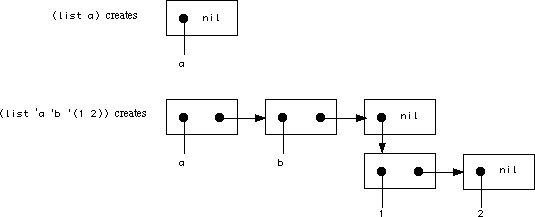• `append` - any number of arguments - all, save the last, must be true lists (and it usually is, too). Combines its arguments into a single list, which will be a true list if the last argument is. For example, `(append '(1 2) '(3 4))` is `(1 2 3 4)`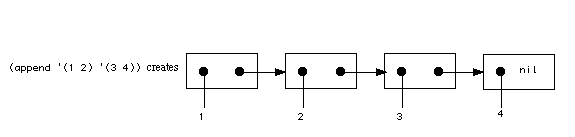### Arithmetic

LISP supports the arithmetic operations found in most programming languages, plus many unique ones as well. Most of the operations take any number of arguments - e.g. `(* 2 3 4 5)` evaluates to 120. All are functions, which means that the arguments are evaluated before the operation is performed.

• `+` addition - any number of arguments; with no arguments gives the identity element for addition, 0.
• `-` negation - one argument; subtraction - two or more arguments; illegal with no arguments.
• `*` multiplication - any number of arguments; with no arguments gives the identity element for multiplication, 1.
• `/` reciprocal - one argument; division - two or more arguments; illegal with no arguments.
• `1+` increment by 1 - one argument only.
• `1-` decrement by 1 - one argument only.
• `exp` e to the power - one argument only.
• `expt` number to the power - two arguments only.

### Tests

These are functions that apply some test one or two values (variables or expressions - evaluated before the test is applied) and either succeed (evaluating to `t`) or fail (evaluating to `nil`).

• `atom` - true if its single argument is an atom (see the type hierarchy above).

• `symbolp` - true if its single argument is a symbol (see the type hierarchy above).

• `numberp` - true if its single argument is a number (see the type hierarchy above).

• `listp` - true if its single argument is a (true or dotted) list (see the type hierarchy above).

• `null` - true if its single argument is the empty list (`()` or `nil`).

• `eq` - true if its two arguments are the same identical object. Because of the way LISP handles symbols, a given name always maps to the same symbol, so something like `(eq 'a 'a)` is always true.

• `eql` - true if its two arguments are `eq` or are numbers with the same type and value or characters with the same value.

• `equal` - true if its two arguments are isomorphic - i.e. their printed representation is the same.

### Comparisons

LISP supports the standard comparisons on numbers, but most can take one up to any number of arguments, rather than just two. (In the case of two arguments, they behave in the traditional way.) All of the arguments must be numbers.

• `=` - all arguments equal

• `/=` - all arguments differ (no two equal)

• `>` - all arguments monotonically increasing

• `<` - all arguments monotonically decreasing

• `>=` - all arguments monotonically non-decreasing

• `<=` - all arguments monotonically non-increasing

• `zerop` - one argument only - true if the argument is 0

### Input-Output

Common Lisp provides a large number of input output operations. Input operations are functions whose value is some input read from an external stream; output operations are typically functions which have the side effect of printing something on an external stream and also return as their function value that which was printed.

• `read` no argument - reads a single s-expression

• `print` single argument - prints a single s-expression, preceeded by a newline and followed by a single space.

• `prin1` single argument - prints a single s-expression, with no whitespace before or after.

• `princ` single argument - prints a single s-expression, with no whitespace before or after, and without special characters such as quotes around a string.

• `terpri` no argument - prints a single newline.